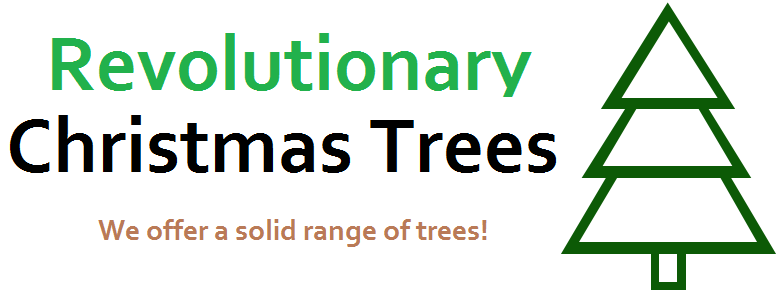# Day 6: Revolutionary Christmas Trees

Calculus Level 2A mathematician is buying a Christmas tree from Revolutionary Christmas Trees.

He sends this model function ( $x$ in terms of $y$ ):

$x = \begin{cases} 2 - ( \frac{\lfloor y \rfloor }{2} + \{ y \} ) & 0 \leq y < 3\\ \frac{1}{2} & -1 \leq y < 0\\ 0 & \text{otherwise} \end{cases}$

This is rotated round the $y$-axis to create a solid of revolution to model his tree.

He then sends a volume enlargement scale factor $s$ by which the volume of the solid is multiplied to make the tree the correct size. He wishes to have a final volume of $640 \pi$.

Find the value of $s$.

Note: The notation {$y$} means the fractional part of $y$

×

Problem Loading...

Note Loading...

Set Loading...